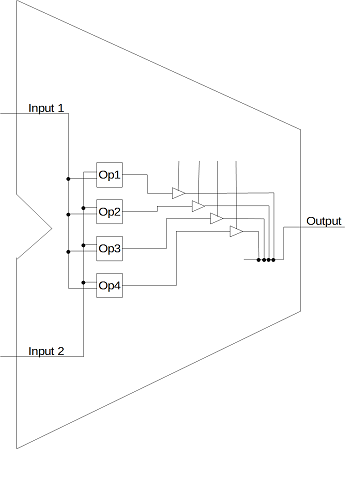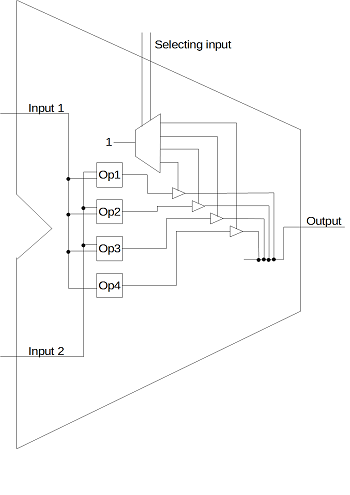# Creating an Assembly Language Using an Instruction Set

Shadi has a Ph.D. in Computer Science and more than 20 years experience in industry and higher education.

An ALU is capable of executing several instructions or different operations, otherwise known as an instruction set. In addition, it includes different functional units, each having its inputs and its outputs. In this lesson, we will see how these different outputs are connected to one output of the ALU, and learn how this leads to machine code and assembly language creation.

## Introduction

A CPU is composed of two main units: 1) the ALU (Arithmetic/Logic Unit) and 2) the CU (Control Unit).

The ALU is the part of the CPU that carries out the arithmetic and logic operations such as addition, increment, logic AND, etc. In general, an ALU has two inputs and one output. Internally, this is implemented by including different functional sub-units inside the ALU. The outputs are connected to the ALU output through 3-state buffers. Figure 1 shows how the output of a 1-bit ALU is implemented using 3-state buffers. Building an n-bit ALU is fairly easy. It is done by duplicating the 1-bit ALU n-times.The CU manages the instruction queue functionality of the CPU. The CU sends the necessary signals to the selection inputs of the ALU. In turn, the ALU outputs one of several operations it is capable of executing.

In this lesson, we are addressing the following design problem: Given that we have an ALU capable of executing multiple functions, how can we choose which one of these functions should be connected to the output of the ALU?

## Codifying the Instruction Set

An instruction set is the set of 'naively-executable' instructions (or simply native instructions) of a CPU. By 'naively-executable' we mean that they are executed directly by the hardware of the ALU and do not require a multi-instruction program to execute them.

To understand how to build an instruction set and then create assembly language out of it, let us work on a simple example. Consider the ALU shown in Figure 1. This ALU is capable of executing 4 native instructions. Suppose that these operations are addition, subtraction, logic AND and logic NOT. The question now is this: How do we choose one of the four outputs to be connected to the ALU output. You got it right! We need a demultiplexer. A demultiplexer is a combinational circuit that connects its data input to one of its outputs, depending on the value of its selecting input. Accordingly, we can add a demultiplexer to the circuit in Figure 1 and we will get the circuit in Figure 2. This circuit adds selection inputs to the ALU.The selection inputs in Figure 2 represent the machine language of the ALU (and the CPU). In other words, let us assume that we will choose the following selection values for the four available operations:

• 00 for the addition operation
• 01 for the subtraction operation
• 10 for the logic AND operation
• 11 for the logic NOT operation

If the ALU receives the value 00 on its selection input, the demultiplexer will pass the value of the addition operation to the ALU's output. If the selection value is 01, the output of the ALU will be the difference between the two inputs.

To unlock this lesson you must be a Study.com Member.

### Register to view this lesson

Are you a student or a teacher?